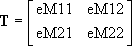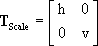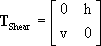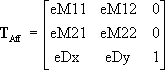# HTML_PAINT_XFORM structure

Represents matrices in the HTML_PAINT_DRAW_INFO structure for transforming or translating a rendering behavior's display output when the behavior supports transformations.

## Syntax

``````typedef struct _HTML_PAINT_XFORM {
FLOAT eM11;
FLOAT eM12;
FLOAT eM21;
FLOAT eM22;
FLOAT eDx;
FLOAT eDy;
} HTML_PAINT_XFORM;
``````

## Members

• eM11
Member of a transformation matrix. For details, see Remarks.

• eM12
Member of a transformation matrix. For details, see Remarks.

• eM21
Member of a transformation matrix. For details, see Remarks.

• eM22
Member of a transformation matrix. For details, see Remarks.

• eDx
Translation amount in the horizontal direction.

• eDy
Translation amount in the vertical direction.

## Remarks

When a rendering behavior has indicated it supports tranformations, MSHTML will supply transformation values in an HTML_PAINT_XFORM structure. This structure is available to the rendering behavior during MSHTML calls to the behavior's implementation of IHTMLPainter::Draw. The behavior retrieves this structure by calling IHTMLPaintSite::GetDrawInfo. (A rendering behavior must specify the HTMLPAINT_DRAWINFO_XFORM flag when calling IHTMLPaintSite::GetDrawInfo to retrieve transformation information.) This method retrieves data to an HTML_PAINT_DRAW_INFO structure that itself contains the HTML_PAINT_XFORM structure.

Note  A rendering behavior indicates that it supports transformations by implementing IHTMLPainter::GetPainterInfo so that it sets the HTMLPAINTER_SUPPORTS_XFORM flag in the lFlags member of the HTML_PAINTER_INFO* pInfo parameter. MSHTML calls IHTMLPainter::GetPainterInfo to determine the rendering behavior's capabilities and requirements. If a rendering behavior does not support transformations, the behavior will not be drawn under some circumstances—when a IHTMLRuleStyle3::zoom style is applied to the region containing the rendering behavior, for instance.

When implementing IHTMLPainter to support transformations, it is not necessary to know the kind of tranformation because MSHTML supplies the values. The implementer's responsibility is to retrieve the structure and add or multiply its values by the x- and y- coordinates being drawn, according to the following formulas.

The HTML_PAINT_XFORM structure offers two ways to alter how a behavior renders its shapes: transformation and translation. Transformations are applied before translation.

Transformations are of four types: scaling, rotation, shear, and reflection.

The four values eM11, eM12, eM21, and eM22 can be thought of as members of a 2 x 2 transformation matrix, T.The vector for the coordinates of the original point is multiplied by this transformation matrix to calculate the coordinates of the transformed point.The kind of transformation—scaling, rotation, shear, or reflection—is determined by the values for eM11, eM12, eM21, and eM22.

• A scaling transformation stretches or shrinks a shape in the horizontal and vertical direction. The general form is shown in the following equation.where h and v are the multipliers for the horizontal and vertical directions respectively. A value greater than 1 enlarges a shape in that direction. A value less than 1 shrinks it.

• A rotation transformation rotates a shape around the origin by a specified angle in a clockwise direction. The general form is shown in the following equation.where a is the angle of rotation.

• A reflection transformation flips a shape around a line, creating a mirror image of the shape on the other side of the line. The general form is shown in the following equation.where w is the clockwise angle between the x-axis and the line of reflection passing through the origin.

• A shear transformation deforms a shape by tilting it over or stretching it into a parallelogram, much like the way italics tilt plain text.The general form is shown in the following equation.Translations shift a shape by specified amounts in the horizontal (eDx) and vertical (eDy) directions. In matrix terms, the values eDx and eDy form a 1 x 2 matrix (or vector) that is added to the vector for the original coordinates to calculate the translated coordinates, as shown in the following equation.Here, x and y are the original coordinates of any point in the shape, and x' and y' are the coordinates for the translated point as it will be rendered.

The tranformation and translation can also be represented as an affine transformation using a single 3 x 3 affine matrix, as shown in the following equation.The following shows the complete equation.## Requirements

 Minimum supported client Windows XP Minimum supported server Windows 2000 Server Header Mshtml.h IDL Mshtml.idl